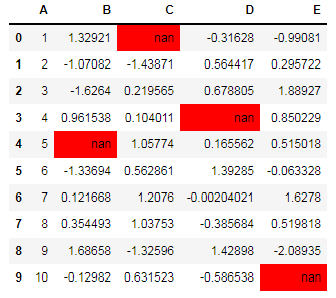﻿ Pandas styling: Write a Pandas program which will highlight the nan values - w3resource# Pandas styling Exercises: Write a Pandas program which will highlight the nan values

## Pandas styling: Exercise-2 with Solution

Create a dataframe of ten rows, four columns with random values. Convert some values to nan values. Write a Pandas program which will highlight the nan values.

Sample Solution :

Python Code :

``````import pandas as pd
import numpy as np
np.random.seed(24)
df = pd.DataFrame({'A': np.linspace(1, 10, 10)})
df = pd.concat([df, pd.DataFrame(np.random.randn(10, 4), columns=list('BCDE'))],
axis=1)
df.iloc[0, 2] = np.nan
df.iloc[3, 3] = np.nan
df.iloc[4, 1] = np.nan
df.iloc[9, 4] = np.nan
print("Original array:")
print(df)
def color_negative_red(val):
color = 'red' if val < 0 else 'black'
return 'color: %s' % color
print("\nNegative numbers red and positive numbers black:")
df.style.highlight_null(null_color='red')
``````

Original array:

```Original array:
A         B         C         D         E
0   1.0  1.329212       NaN -0.316280 -0.990810
1   2.0 -1.070816 -1.438713  0.564417  0.295722
2   3.0 -1.626404  0.219565  0.678805  1.889273
3   4.0  0.961538  0.104011       NaN  0.850229
4   5.0       NaN  1.057737  0.165562  0.515018
5   6.0 -1.336936  0.562861  1.392855 -0.063328
6   7.0  0.121668  1.207603 -0.002040  1.627796
7   8.0  0.354493  1.037528 -0.385684  0.519818
8   9.0  1.686583 -1.325963  1.428984 -2.089354
9  10.0 -0.129820  0.631523 -0.586538       NaN

Negative numbers red and positive numbers black:
```

Sample Output:

``````

Python Code Editor:

Have another way to solve this solution? Contribute your code (and comments) through Disqus.

What is the difficulty level of this exercise?

﻿

## Python: Tips of the Day

Returns the maximum value of a list, after mapping each element to a value using the provided function

Example:

```def tips_max(lst, fn):
return max(map(fn, lst))

print(tips_max([{ 'n': 4 }, { 'n': 2 }, { 'n': 8 }, { 'n': 6 }], lambda v : v['n']))
```

Output:

```8
```